Highlight Detection，直译过来就是高光检测，一般应用在图像或视频里，本文主要关注视频场景，其任务就是从一段长视频里找到某个“高光”的片段。这里的“高光”是一个非常宽泛的定义，不像 ctr/cvr 有明确的含义，不同场景下对“高光”的定义不一样：比如说对于带货直播，高光片段也许是 gmv 最高的时间段；对于非带货直播，高光的片段也许是观看人数或者刷礼物最多的时间段。

Highlight Detection 在实际的应用场景较为广泛：一些视频网站(如爱奇艺、哔哩哔哩)里鼠标停留在视频上时会自动播放一些片段，这些片段可认为是高光片段；主流的直播平台基本都提供了直播回放工具，其中往往也会提供高光片段的候选，除了提供给用户侧，广告主/商家侧也会提供类似产品，如巨量千川磁力金牛等平台的产品

Highlight Detection 在学术界也是一个研究方向，但是学界基本研究局限在几个人工标注的数据集上，一般无法直接应用到实际的生产环境中，原因就是上面说的，不同场景下对高光的定义不一样，需要的数据集也不一样。Highlight Detection 相关 paper 不少，本文主要讲2 篇更贴近业界的 paper，可以重点关注高光的监督信号的定义，损失函数的设计以及数据集的获取

## TaoHighlight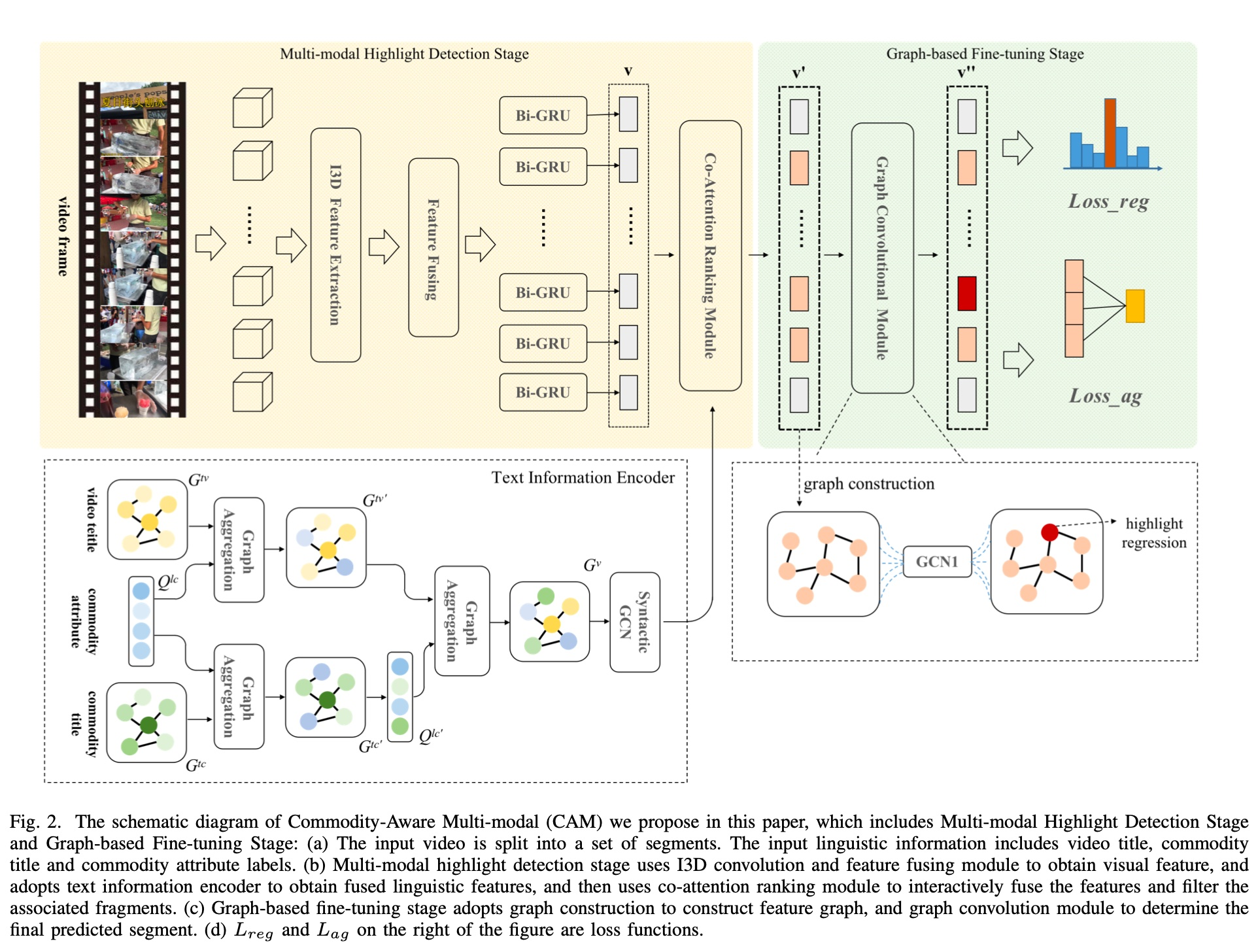Co-Attention Module，这个模块主要作用是融合多模态特征（即 visual information 和 text information），基本的原理是参考了 trasformer 的 self-attention 机制, 对于下面左边的 block，$v$ 相当于 query, $s$ 相当于 key 和 value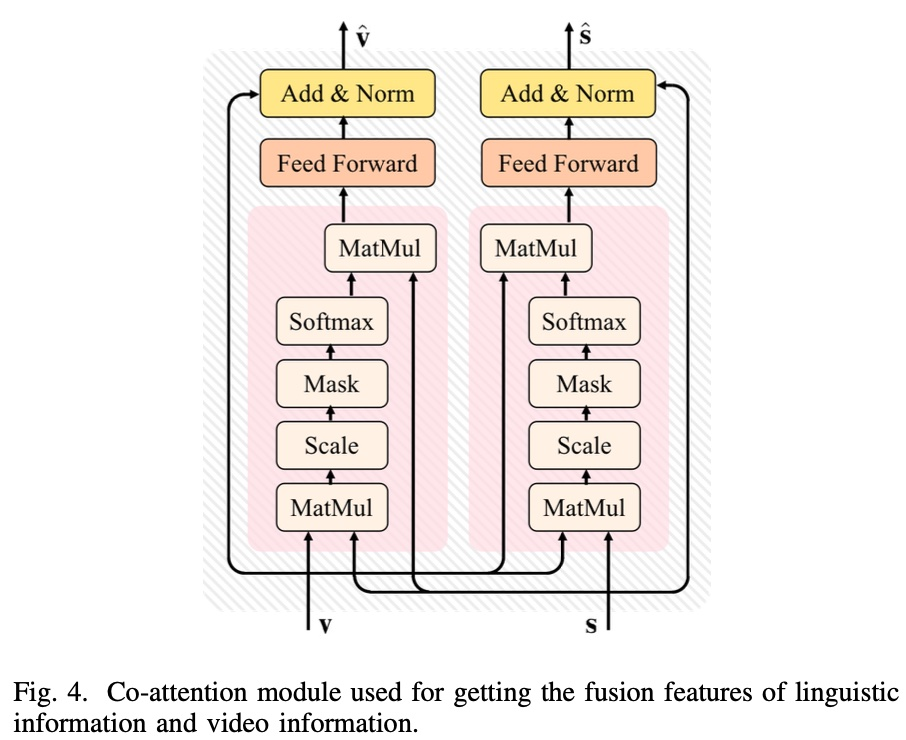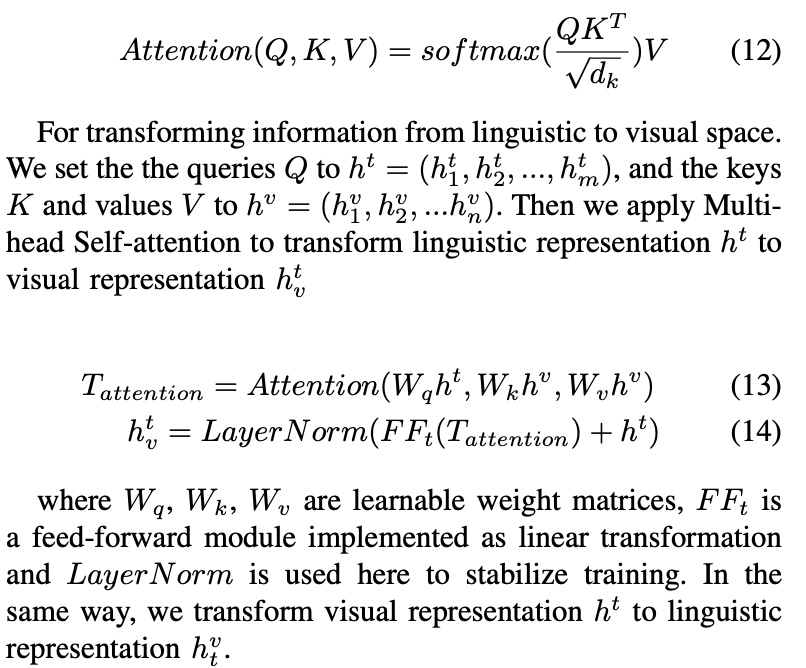Graph-based Fine-tuning: 这部分主要是为了减少抽取出来的特征里的 noise；paper 里是这么说的: Due to the presence of visual and text noises in multi-modal video highlight detection, we propose a graph based fine-tuning module to improve the accuracy of our model.，但是也没进一步说明原因

$L_{reg}$ ，计算预估的开始/结束时间和真正的开始/结束时间的的差异，计算方式如下

$$L_{reg} = \frac{1}{N} \sum_{i=1}^{N}[R(\hat{s_i}, s_i) + R(\hat{e_i}, e_i)]$$

• $s_i$, $e_i$: 预估的高光片段的开始和结束时间点
• $\hat{s_i}$, $\hat{e_i}$: 高光开始和结束时间的 ground truth
• $R$: L1 函数

$L_{ag}$ 计算方式如下, 主要用来计算两段视频的相关性，$k$ 表示将每段视频切成 $k$ 段 clips，主要由三项组成

$$L_{ag} = - \sum_{i=1}^{k}e(v_i, \hat{v})$$

$$e(v_i, v_j) = \theta_{r} \cdot r(v_i, v_j)+\theta_{d} \cdot d(v_i, v_j)+\theta_{s} \cdot \cos(v_i, v_j)$$

• $v_i$, $\hat{v_i}$: 预估的高光片段和 ground truth
• $r(v_i, v_j) = \frac{I(v_i, v_j)}{U(v_i, v_j)}$, 就是 IoU 指标，表示重合面积占比
• $d(v_i, v_j) = \frac{ |c_i - c_j|}{U(v_i, v_j)}$, $c_i$ 和 $c_j$ 表示两个 video 的中心位置
• $cos$: 两个片段的 cos 相似性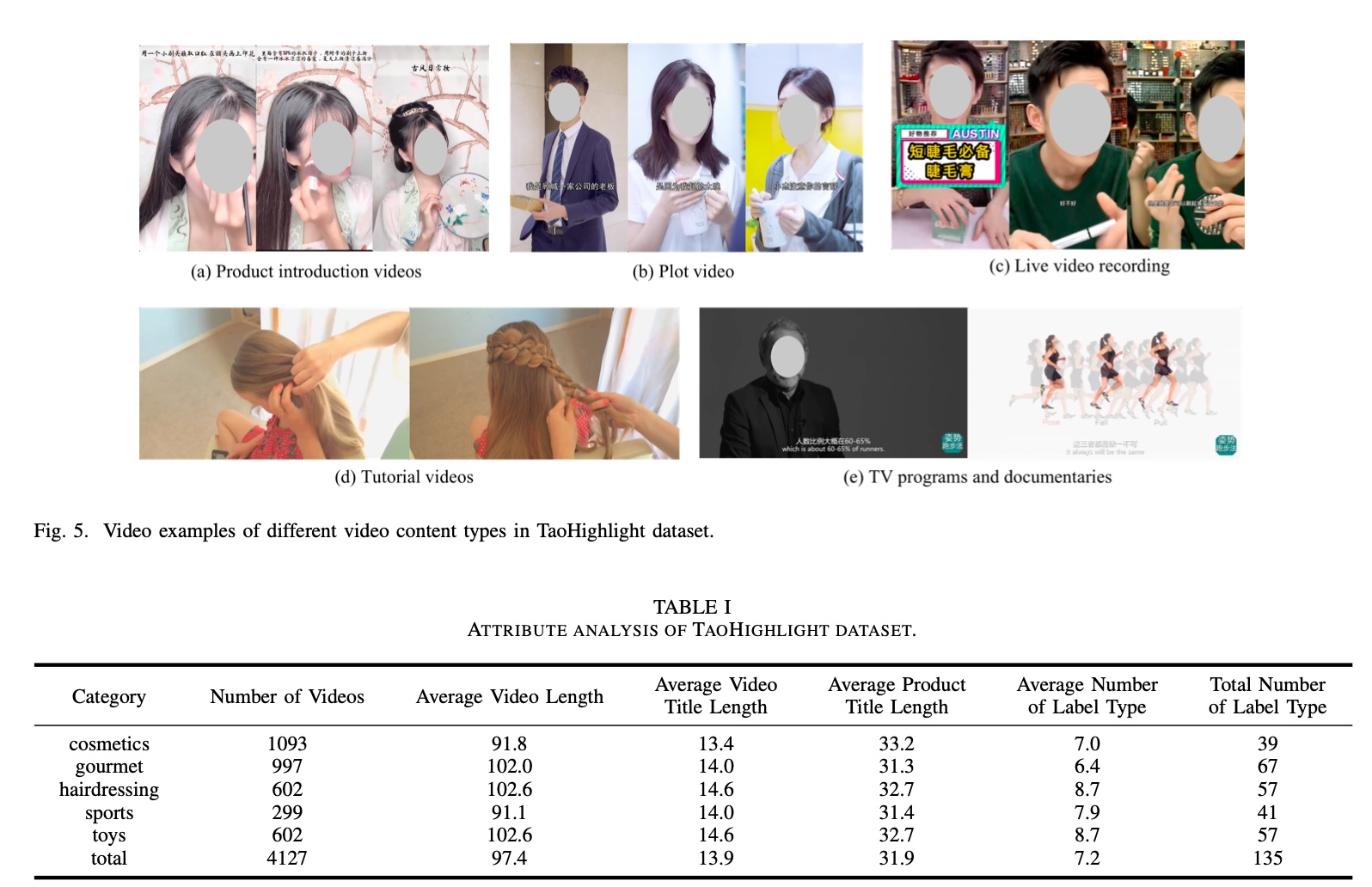paper 做了消融实验，结果如下图所示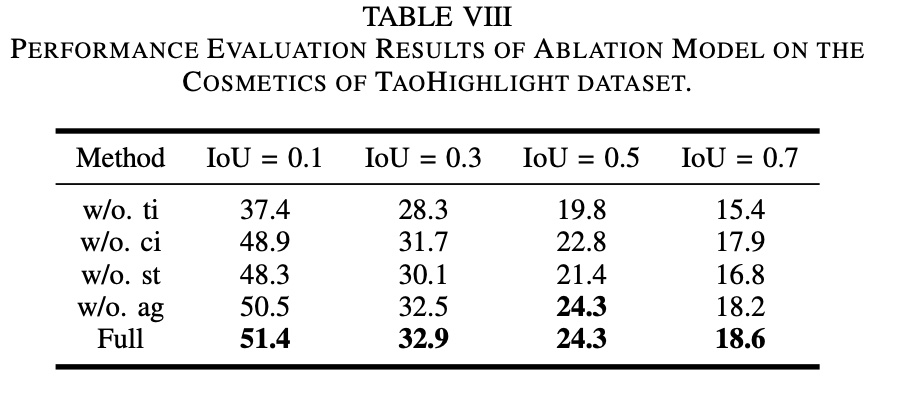• w/o.ti. 去掉文本特征
• w/o.ci. 文本特征只包含 video title（去掉了商品 titile 和商品属性）
• w/o.st. 去掉了 Graph-based Fine-tuning
• w/o.ag. 去掉了损失函数中的 $L_{ag}$ 项

## “unsupervised” solution

peper 认为 Less is More, 即越短的视频的信息量就越高，所以切分出来的片段都可以认为是高光片段，反之越长的视频的信息量约低，切出来的都不是高光片段，因此，paper 将训练样本 $D$ 分为分为三部分，即$D= \lbrace D_S, D_L, D_R \rbrace$，$D_S$ 表示短视频的集合，$D_L$ 表示长视频的集合，paper 将短于 15s 定义为短视频，长于 45s 的定义为长视频

paper 采用了 pair-wise 的方法来构造样本，即从 $D_S$ 和 $D_L$ 切好的 segment 中分别取出一个，来构成一对 pair $(s_i, s_j)$，然后基于下面的 ranking loss 计算两部分的差异，这里的 ranking loss 其实是一类损失函数，常见的 triplet loss、magrin loss、hinge loss 其实都可以算做 ranking loss

$$L(D) = \sum_{(s_i, s_j) \in \mathcal{P}} \max(0, 1 - f(x_i) + f(x_j))$$

\begin{align*} &L(D) = \sum_{(s_i, s_j) \in \mathcal{P}} w_{ij} \max(0, 1 - f(x_i) + f(x_j)) \\ &\begin{array} \\ s.t.& \sum_{(s_i, s_j) \in \mathcal{P}} w_{ij} = p|\mathcal{P}|, w_{ij} \in [0,1] \\ &w_{ij} = h(x_i, x_j) \end{array} \end{align*}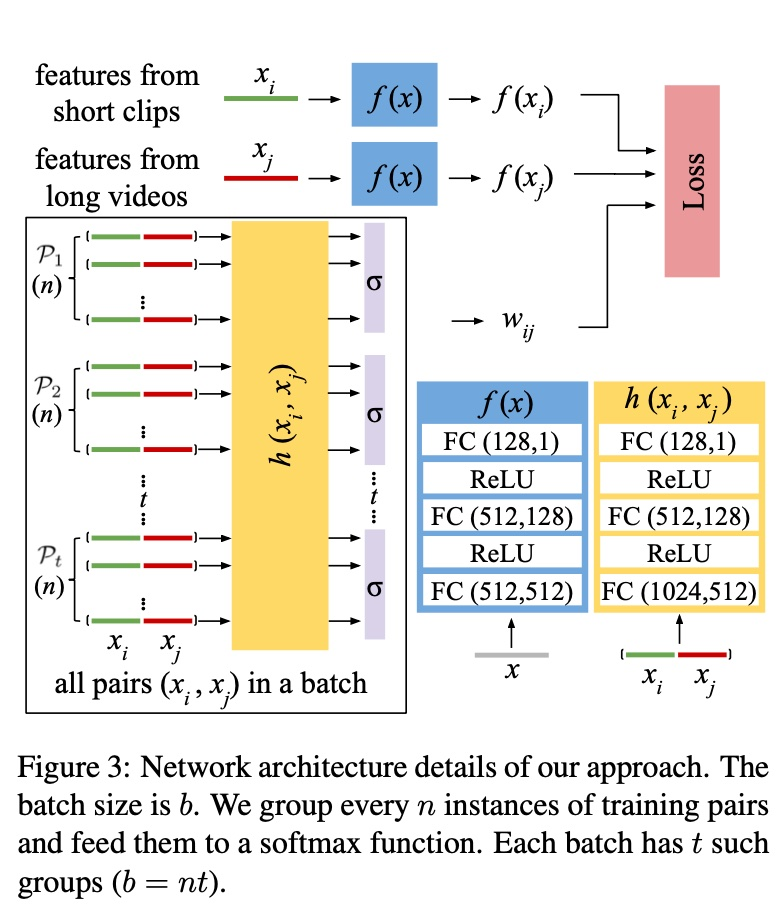\begin{align*} &L(D) = \sum_{g=1}^{m} \sum_{(s_i, s_j) \in \mathcal{P_g}} w_{ij} \max(0, 1 - f(x_i) + f(x_j)) \\ &\begin{array}\\ s.t.& \sum_{(s_i, s_j) \in \mathcal{P_g}} w_{ij} = \sum_{(s_i, s_j) \in \mathcal{P_g}} \sigma(h(x_i, x_j)) = 1 \\ &w_{ij} \in [0,1] \end{array} \end{align*}

mAP 的定义跟 AUC 有点像，只是这里采用了 PR 曲线，AUC 采用的是 ROC 曲线，两者的区别可参考 ROC 曲线与 PR 曲线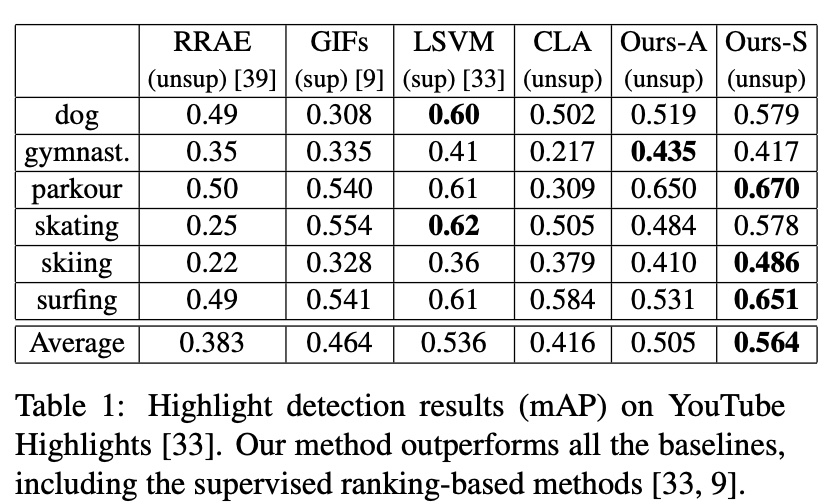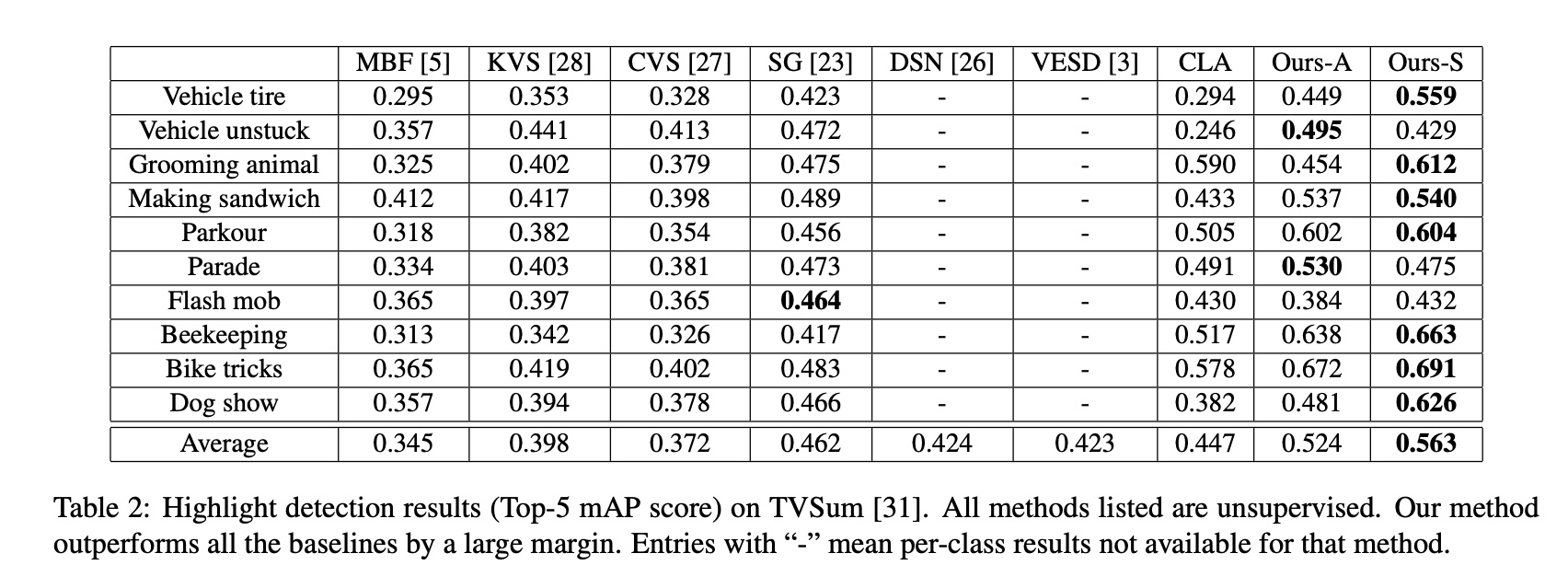（1）针对上面的binary latent variable $w_{ij}$，对比了去掉 $w_{ij}$ （下图中的Ranking-D）和通过 EM 来更新 $w_{ij}$ （下图中的 Ranking-EM）的效果，效果是 joint training > EM > 去掉 $w_{ij}$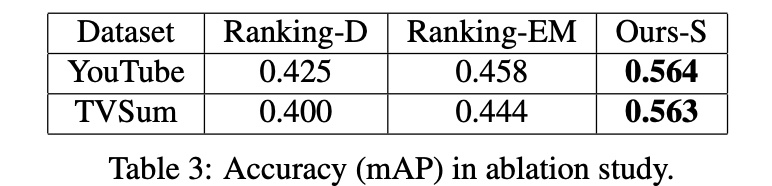（2）对比了数据集大小的影响，随着数据集增大，准确率逐渐上升并减缓，比较常规的结论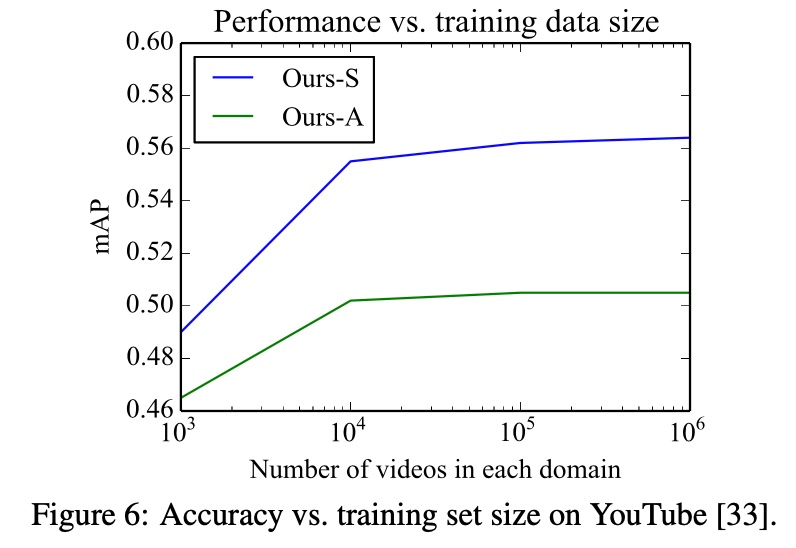## 小结

• 特征工程: video 和 text 特征的提取，通过 co-attention 机制融合这两部分特征
• 损失函数的设计，$L_{reg}$ + $L_{ag}$
• 减少noise: graph-based fine-tunning 模块，对一些 topk 的候选做 fine-tuning

• 数据集，根据 video 的长短来判断视频的是否属于高光，无需人工打标
• 损失函数的设计，pair-wise 的 ranking loss
• 减少noise: 通过一个可训练的 binary latent variable 来标识样本的置信度

1. video duration 是一个比较粗糙的信号，实际的业务中，会有很多的指标(比如说 ctr、cvr、roi等)，这些指标作为高光的定义也许是一个更好的选择，同时需要权衡选择的信号的深度和数据稀疏的 trade-off
2. 除了以上的 ranking loss，LTR(pair-wise, list-wise) 建模也是一个不错的选择，实际业务中需要考虑 pair 或 list 怎么构建
3. 如果是直播的场景下，需要实时做高光的检测，无法拿到整个视频，需要考虑一种流式的检测方法